KG1.1
 Name:    KG1.1

Multiple Choice
Identify the choice that best completes the statement or answers the question.

1.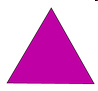What shape is this?
 a. rectangle c. square b. sphere d. triangle

2.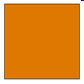What shape is this?
 a. rectangle c. square b. sphere d. triangle

3.

Which object below is a sphere?
 a.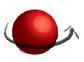c.b.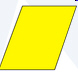4.

Which object below is a cone?
 a.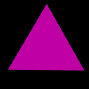c.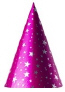b.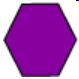5.

Which object below is a cylinder?
 a.c.b.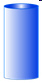6.

Which object below is a hexagon?
 a.c.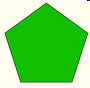b.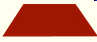7.

Which object below is a cube?
 a.c.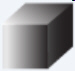b.